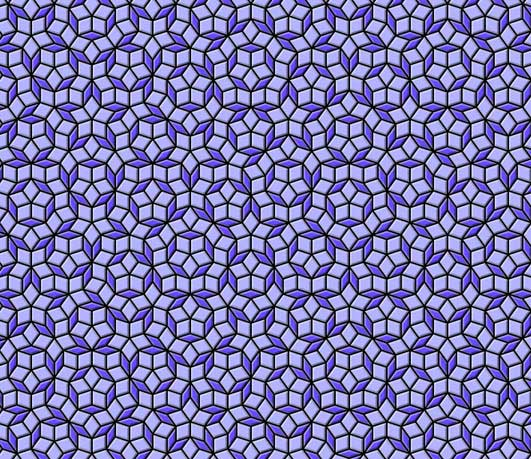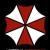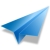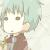谁说使用Python你就写不出混乱的代码？

CIIS 2019丨CIIS 2019丨倒计时10天丨CIIS 2019 嘉宾阵容公布！>>>_                                 =\
"""if!
1:"e,V=100
0,(0j-1)**-.2;
v,S=.5/  V.real,
[(0,0,4      *e,4*e*
V)];w=1          -v"def!
E(T,A,              B,C):P
,Q,R=B*w+                A*v,B*w+C
*v,A*w+B*v;retur              n[(1,Q,C,A),(1,P
,Q,B),(0,Q,P,A)]*T+[(0,C            ,R,B),(1,R,C,A)]*(1-T)"f
or!i!in!_[:11]:S       =sum([E          (*x)for       !x!in!S],[])"imp
ort!cair               o!as!O;      s=O.Ima               geSurfac
e(1,e,e)               ;c=O.Con  text(s);               M,L,G=c.
move_to                ,c.line_to,c.s                et_sour
ce_rgb                a"def!z(f,a)                :f(-a.
imag,a.       real-e-e)"for!T,A,B,C!in[i       !for!i!
in!S!if!i[""";exec(reduce(lambda x,i:x.replace(chr
(i),"\n "[34-i:]),   range(   35),_+"""0]]:z(M,A
);z(L,B);z         (L,C);         c.close_pa
th()"G             (.4,.3             ,1);c.
paint(             );G(.7             ,.7,1)
;c.fil             l()"fo             r!i!in
!range             (9):"!             g=1-i/
8;d=i/          4*g;G(d,d,d,          1-g*.8
)"!def     !y(f,a):z(f,a+(1+2j)*(     1j**(i
/2.))*g)"!for!T,A,B,C!in!S:y(M,C);y(L,A);y(M
,A);y(L,B)"!c.st            roke()"s.write_t
o_png('pen                        rose.png')
"""                                       ))最新评论（14）【图案呈不规则周期状分布——你移动角度后都找不到再次重合的图案。】——不知道这句话怎么得来的，就新闻里这个局部的图，就有3出图案是一样的！NB挺挺啊。。。引用来自“霸气千秋”的评论

+1